# The sphere-cone equation in a matrix notation.

It is about time for a new post on 3D numbers, circular and complex. In this post I write the sphere-cone equation in a matrix notation so see the previous post on conjugates if you feel confused. The sphere-cone equation gives us two equations, as the name suggests these are a sphere and a cone and on the intersection we find the famous exponential circle.

Beside the sphere-cone equation I also demand that the determinant equals 1, now we have three equations and every intersection of those 3 equations has as it’s solution the exponential circle. Can it become more crazy? Yes because it is possible to factorize the third degree determinant into a linear and a quadratic factor. Those factors must also be 1 and now we have five equations! And since you can pick 10 pairs out of five, we now have 10 ways of solving for the intersection where the exponential circle lives…

It is strange that after all these years it is still easy to find 10 video’s where so called ‘professional math professors’ sing their praise upon the exponential circle in the complex plane. They really go beserk over the fact that e to the power it gives the cosine and sine thing. And after all those years still silent, yeah yeah those hero’s really deserve the title of honorable shithole… It is honorable because they often have relatively large salaries and they are shitholes because of their brave behavior when it comes to 3D complex numbers. Bah, I am getting a bad taste in my mouth when I think about the behavior of professional math professors. Let me stop writing about that low form of life.

This post is 8 pictures long. May be, I have not decided yet, is the next post about parametrizations of the exponential circle. In these 8 pictures I work out the case for the circular multiplication, that is the case where the imaginary unit j behaves like j^3 = 1. At the end I only give the 3D complex version of the matrix form of the sphere-cone equation and the rest you are supposed to do yourself.

Ok, again do not confuse this with quadratic forms. A matrix equation as written above has a real and two imaginary components while quadratic forms are often just real valued.

Let´s try to upload this stuff. See you in the next post.

# The two self-conjugate planes for 3D circular and complex numbers.

This is another lightweight easy going summer update. It is about matrix representations and how to find the conjugate of a 3D complex or circular number. I use the case of the complex plane of 2D conplex numbers to show that conjugation is not some silly reflection just always but rather simple will always be the upper row of a proper matrix representation. As a matter of fact it is so easy to understand that even the biggest idiots on this planet could understand it if they wanted. Of course math professors don’t want to understand 3D numbers so also this new school year nothing will happen on that front…

Did you know that math professors study the periodic system? Yes they do, anyway in my home country the Netherlands they do because every year they get a pay rise and that pay rise is called a periodic. And as such they study the periodic system deep and hard…

I classified this post only under the categories ‘3D complex numbers’ and ‘matrix representations’ and left all stuff related to exponential circles out. Yet the exponential circle stuff is interesting; after reading this post try to find out if the numbers alpha (the midpoint of the exponential circles) are symmetrix (yes). And the two numbers tau (the log of the first imaginary unit on the circular and complex 3D space) are anti-symmetrix (yes).

This post is just over 7 pictures long. As the background picture I used the one I crafted for the general theorem of Pythagoras. (Never read that one? Use the search funtion for this website please!) All pictures are of the usual size namely 550×775 pixels.It is a cute background picture, I remember it was relatively much work but the result was fine.

Ok, that was it for this update. Although it is so very simple (for years I did not want to write of just two simple planes that contain all the self-conjugate numbers) but why make it always so difficult? Come on it is summer time and in the summer almost all things are more important than math. For example goalkeeper cat is far more important compared to those stupid 3D numbers. So finally I repost a video about a cat and that makes me very similar to about 3 billion other people.

# But are these quadratic forms?

This is a lazy easy going summer post, it does not have much mathematical depth. Let’s say the depth of a bird bath. But with most posts I write you also need a lot of knowledge about what was in previous posts and for the average person coming along that is often too time consuming… So we keep it simple today; quadratic forms on 3D space.

If you have had one or two courses of linear algebra you likely have encountered quadratic forms. They are often denoted as Q(X) where the X is a column matrix and the quadratic form is defined as Q(X) = XT A X. Here XT is the transponent of X so that would be a matrix row. As you might guess, the X column matrix contains the variables while the constant square matrix A is the source of coefficients in the quadratic form Q(X).  In most literature it is told the matrix A is symmetric, of course there is no reason at all for that; any square matrix will do. On the other hand it is easy to see or to show that if a square matrix is anti-symmetric the corresponding quadratic form will always be zero everywhere.

In this post we will take matrices that are always the matrix representation of 3D complex & circular numbers. Matrix representations are a complete category on this website so if you don’t know them you must look that up first. (Oh oh, here I go again: this was supposed to be easy but now the average reader must first try to understand matrix representations of higher dimensional multiplications…)

Compared to the previous update on the likely failure of all fusion reactors this post is far less dramatic. If in the future I am right and we will never have fusion power, that will be the difference between life and death of hundreds of millions of people in the long run… So in order to be a bit less depressing let’s lift the spirits by a lightweight new post on quadratic forms! Why not enjoy life as long as it lasts?

Ok, the actual post is seven pictures long, all in the usual size of 550×775 pixels.

I have to admit that for me the use of the number alpha was important because that is at the center of the exponential circles in the 3D complex and circular spaces. So I have a legitimate reason to post this also under the category ´exponential circle´. And from the non-bird bath deep math, that is the big math ocean that is very deep, I like to classify as much posts under that category ´exponential circles´.

Ok, let´s leave it with that and try to upload this post. Till updates my dear reader.

# New roots of unity (the 3D complex ones) & The rain theorem.

I just finished brewing the 100-th batch of a beer under the names Dark Matter and Spin 1/2 beer. All in all that is an amazing amount of beer; in the past I brewed 35 liter per batch but now it is 40 liter per batch so all in all an amount of something between 3500 and 4000 liters… So ein prosit my dear reader.

The ‘new’ roots of unity aren’t that new, this post is a re-editing of something I posted on 05 Jan 2014 on the other website. Later that year I started this website. Actually these roots of unity are just over five years old. In mathematical terms that is still very young so in that sense they are still new.

Recall the roots of unity in the complex plane are solutions to z^n = 1 and as such these roots are found on the exponential circle (the complex exponential) in the complex plane. As you have found the exponential circle or exponential curve in some space, from that you can always make new roots of unity. That is hardly a mathematical achievement because it is so simple to do once you have found your exponential circle or curve. But in the diverse spaces these new roots of unity behave very different, for example in this post we will add them all up but unlike in the complex plane they do not add up to zero. That is caused by the fact that in the complex 3D space the number alpha is at the center of the exponential circle, as such if we add n roots of unity in 3D space the result is n times alpha. Last year we studied the space of 4D complex numbers and if you would craft new roots of unity in that space it will behave much more like those in the complex plane because in the 4D complex numbers we have 0 as the center of the exponential curve. (For dimensions above 3 the exponential curve always lies in a hyperplane so it can never be a circle.) It always amazes me that you have all those physics people who study string theory but as far as I know never use exponential curves…

Life is beautiful, because how can you do string theory without math like that? But in physics almost everything is beautiful, for example if they explain the outcome of the Stern-Gerlach experiment always 50% of unpaired electrons align with the applied magnetic field and the other 50% for some mysterious reason do the anti-align thing. And if one hour later the same physics professor explains how a permanent magnet can attract some piece of iron, all of a sudden 100% of the unpaired electrons align and all that talk of 50/50 suddenly is not observed… Life inside the science of physics is wonderful; all you have to do is a bit of blah blah blah and if people complain this is not logical at all you simply say: Quantum physics is such that if you think you understand it, you don’t understand it… How wonderful is the life of physics professors; talk some blah blah blah and if people complain you blame them for ‘not understanding quantum things’. For sure that is a beautiful form of life.

But enough of the talking, somewhere in the next seven pictures I did forget to insert a graph of the determinant. Yet I showed you the structure of the non-invertible numbers so often, I think I post it with that fault included. After all why should life be perfect? If life would be perfect you would have no way of improvement and likely that is the moment you die: no more possibility of improvement. As usual the pictures are 550×775 pixels but I had to make the first one a tiny bit longer. Good luck with digesting it & have a bit of fun in the process.

For myself speaking it was fun to read my own two proof for the rain theorem again after five years. Please do not forget that new roots of unity on other spaces can be very different in behavior, after all they are always part of the exponential circle or curve in that space so they will derive their math properties from that. Till updates.

# Short intro to the rain theorem.

At first I wanted the next post to be about the so called Bell experiment because with this experiment comes the so called Bell inequalities and weirdly enough these are a perfect for the determinant of 4D complex numbers. Everything just looked perfect: the Bell inequality has maximum breaching when a bunch of correlations takes on the value of two time the square root of two. But that value of two time the square root of two is also what makes a 4D complex number on the unit sphere in 4D space noninvertible… So everything looked perfect but in the end it did not work because I could only find solutions with correlations above 1 (or below -1) and we all know that is not possible.

So let’s put the stuff on the shelf and wait a few years… The Bell experiment is one of those crazy quantum experiments and I am very interested in it because the original proposition as done by Mr. Bell was done with electrons and positrons. Yet results with electrons and positrons have never been published, all there is are experiments with photons and all those experiments seem to violate the Bell inequality…

Anyway a few days back I came across some old work I had written and that contained stuff like the anihilation theorem and the rain theorem, I did absolutely not remember what it all was about. And oh yes, it was that time that I wrote about a new set of so called roots of unity. And I remembered that I more or less hoped all those years ago about some kind of reaction from the math professionals. Of course there was zero reaction one more year; in those long lost years I still had to learn that university math professors are all shit. That is a uniform property of those people; they are all perfumed princes in relatively high paid permanent jobs. And perfumed princes do what the average perfumed prince think is important: we do perfumed prince stuff like the Langlands program and oh oh oh how smart we are. Luckily we have no dealings with those dirty peasants that live in the mud and cannot afford our exclusive perfumes. Tax payers should be happy they can finance us because what is a modern economy without smart math professors?

Once more I was stupid to the bone: The fact that you can easily find 10 videos with math professionals stating that the roots of unity are so very wonderful does not mean if you throw in a new set of unity roots there will be a healthy response…

I will leave the new post more or less like the old one, I only change the title and will do some editing to make it more readable. For example in the past I used the matrix environment for multi-line calculations while at present day I use the align command in the Latex typesetting program.

Here is the old file from five years back:

http://kinkytshirts.nl/rootdirectory/just_some_math/3d_complex_stuff02.htm#05Jan2014

Ok, a small screen shot of the new roots of unity. Actually they are already five years old but the perfumed math professors have of course better things to do. They are so smart…As you see it could use a bit typo improvement, in the meantime let it rain perfume.

End of the intro to the rain theorem.

Ok, I have done editing and decided to make a very simple teaser picture containing a simple calculation that indeed shows that if you square the opposite point of 1 on the exponential circle you get +1.
As such we have three solutions to the equation X^2 = 1 in the complex 3D space: the usual X = +1 and -1 and the third solutions lies on the exponential circle. Here is the teaser picture and likely later this week I will hang in the rest on this website.

# 3D numbers: Decomposition of all numbers into two non-invertible numbers.

I got the idea for this post already a couple of years back but I shelved it because I would like to have some application for it. But I still haven’t found a killer application yet anyway I decided to write this rather simple post about the projections you get when you multiply any 3D complex and circular numbers with the number alpha. If you need a refreshment on the importance of the number alpha (or what it actually is) please use the search function of this website and search for ‘Seven properties of the number alpha’.

Now two months back I observed some guy in a video explaining the math you need for quantum physics (yes I have a very boring life) and he was explaining you also need projectors P such that P^2 = P meaning that if P is some measuring operator, if you measure it twice you get the same result. And ha, now I write this post down I realize I did not prove that for both operators in the pictures below so that is something you can do for yourself if you want that.

Basically it goes as next: Pick any 3D number X, circular or complex, and multiply it by the number alpha. The result is a number on the main axis of non-invertible numbers (and as such an entire 2D plane gets projected on each of the main axis non invertible numbers). The other operator is (1 – alpha) and if you multiply any 3D number X by that, it gets projected on the plane of non-invertible numbers (and as such a line gets projected on a point of that plane).

All in all it is very basic, but ha ha ha I am doing this stuff now for years on a row and may be for the average reader it is all not so basic. This post is easier to grasp if you understand the shape of the non-invertible numbers: it is a plane and perpendicular on that plane the main axis and both the plane and main axis go through zero. In this post I skipped all things eigenvalue, but in 3D space we have 3 eigenvalues per capita number so unlike in 4D space we cannot have eigenvalue pairs only. In 3D space it has to be different and that explains more or less the shape of the non-invertible numbers.

This post is five pictures long, as usual all 550×775 pixels and I really hope it is not that hardcore this time.

Before we split, on the other website I posted reason number 73 as why electrons cannot be magnetic dipoles. I was that lucky to come across an old 1971 translation of some stuff of the Goudsmit & Uhlenbeck guys. I always suspected there had been some very sloppy physics going on back in the time at the local Leiden university. The translation confirms that more or less (anyway in my view it does). Even after reading the 1971 translation for a third time I kept on falling from one amazement into the other. Have fun reading it, here is a link:

09 May 2019: Reason 73: In his own words; S. Goudsmit on the discovery of electron spin.

Ok, that was it for this post. Thanks for your attention (even if you are one of those sleazeballs from the Leiden university).

# Using the Cayley-Hamilton theorem to find ‘all’ multiplications in 3D space.

It is a bit vague what exactly a multiplication is, but I always use things that ‘rotate over the dimensions’. For example on the 3D complex space the imiginary unit is written as j and the powers of j simply rotate over the dimensions because:

j = (0, 1, 0)
j^2 = (0, 0, 1) and
j^3 = (-1, 0, 0). Etc, the period becomes 6 in this way because after the sixth power everything repeats.

In this post we will look at a more general formulation of what the third power of j is. The Cayley-Hamilton theorem says that you can write the third power of 3 by 3 matrices always as some linear combination of the lower powers.

That is what we do in this post; we take a look at j^3 = a + bj + cj^2. Here the a, b and c are real numbers. The allowed values that j^3 can take is what I call the ‘parameter space’. This parameter space is rather big, it is almost 3D real space but if you want the 3D Cauchy-Riemann equations to fly it has to be that a is always non zero. There is nothing mysterious about that demand of being non zero: if the constant a = 0, the imaginary unit is no longer invertible and that is the root cause of a whole lot of trouble and we want to avoid that.

It is well known that sir Hamilton tried to find the 3D complex numbers for about a full decade. Because he wanted this 3D complex number space as some extension of the complex plane, he failed in this detail and instead found the quaternions… But if the 3D numbers were some extension of the 2D complex plane, there should be at least one number X in 3D such that it squares to minus one. At the end I give a simple proof why the equation X^2 = -1 cannot be solved in 3D space for all allowed parameters. So although we have a 3D ocean of parameters and as such an infinite amount of different multiplications, none of them contains a number that squares to minus one…

I gave a small theorem covering the impossibility of solving X^2 = -1 a relative harsh name: Trashing the Hamilton approach for 3D complex numbers. This should not be viewed as some emotional statement about the Hamilton guy. It is just what it says: trashing that kind of approach…

This post is 7 pictures long, each of the usual size of 550×775 pixels.

Sorry for the test picture, but the seven jpg pictures refused to upload. And that is strange because they are just seven clean jpg’s. Now it is repaired although I do not understand this strange error.

Anyway have a cool summer. Till updates.

# The Cayley-Hamilton theorem neglected for 25 years?

That is strange, if you don’t know the Cayley-Hamilton theorem; it is the finding that every square matrix A, if you calculate the characteristic polynomial for the matrix A it is always zero. At first this is a very surprising result, but it is easy to prove. It’s importance lies in the fact that in this way you can always break down higher powers of the matrix A in lower powers. In the study of higher dimensional complex and circular numbers we do this all the time. If in 3D space I say that the third power of the imaginary component is minus one, j^3 = -1, we only write the third power as a multiple of the zero’th power…

In this post I will give two simple proofs of the Cayley-Hamilton theorem and although in my brain this is just a one line proof, if you write it down it always gets longer than anticipated.

At the end I show you an old video from the year 1986 from the London Mathematical Society where it is claimed that the CH theorem was neglected for 25 years. Now Hamilton is also famous for having sought the 3D complex numbers for about a full decade before he gave up. And I still do not understand why Hamilton tried this for so long but likely he wanted to include the imaginary unit i from the complex plane in it and that is impossible. Or may be he wanted a 3D complex number system that is also a field (in a field all elements or numbers that are non-zero have an inverse, in algebra wordings; there are no divisors of zero). A 3D field is also impossible and in this post I included a small proof for that.

Furthermore in this post at some point may be you read the words ‘total incompetents’ and ‘local university’. You must not view that as some emotional wording, on the contrary it is a cold clinical description of how math goes over there. So you must not think I am some kind of frustrated person, for me it is enough that I know how for example to craft a 3D complex number system. If they don’t want to do that, be my guest. After all this is a free country and we also have this concept of ‘academic freedom’ where the high shot math professors can do what they want.

And what is this ‘academic freedom’ anyway? If for example unpaired electrons are never magnetically neutral but electron pairs always are magnetically neutral, can the physical reality be that electrons are magnetic dipoles? Of course not, that is a crazy idea to begin with. But 97 years of academic freedom since the Stern-Gerlach experiment have never ever brought any meaningful understanding of the magnetic properties of the electron. If it acts as a magnetic charge and you say it is not a charge it is easy to understand how you can fool yourself for about one century of time.

This post is seven pictures long although the last picture is empty.
The two proofs of the Cayley/Hamilton theorem is how I would prove such a thing but good theorems always have many proofs. All pictures are of the size 550×775 pixels.

Here is the old video from 1986 where it is claimed the Cayley-Hamilton theorem was neglected for about 25 years. Oh oh oh what a deep crime. But the human mind is not made to produce or understand math, so in my view 25 years is a short period of time if in the good old days math professors were equally smart as the present day math professors. The title of the video is The Rise and Fall of Matrices.

Ok let me leave it with that an not post a link to the top wiki on the Cayley-Hamilton theorem where all kinds of interesting proofs are given. Till updates my dear reader.

# I am innocent, I did not do it. I just found the numbers tau in the Schrödinger equation your honour…

Judge: But you were caught red handed placing the number tau in a Schrödinger equation while you do not qualify for being a member of the most bright and enlightened persons in our society: The PHYSISCS PROFESSORS.

Reinko: But Judge I can explain, it was that evil guy that Gerard ‘t Hooft who did it. I can prove that because it is on video.

Judge: Yes you already told that into the statements you made after arrest by the police. So we took the freedom and ask Mr. Gerard ‘t Hooft himself about the evil you have done with molesting the Schödinger equation. Mr. ‘t Hoofd said it had to be Hermitian and although I do not know what that means he said that by using anti-Hermitian matrices you, Reinko Venema, you are nothing more as some sadistic pedophile piece of shit.

Reinko: But judge, it is not Hermitian, that is only a trick. You see if you multiply it by the number 1 like 1 = – i squared you see it is not Hermitian.

Judge: Do you think we get complex analysis in law school? We don’t, we asked some experts and all agreed that Gerard is right and you are wrong and right now rewarded by your own evil deeds to 75 years in prison in a maximum security facility.

__________

After this somewhat strange introduction I repeat I was innocent. I was just looking at a video of a guy that is just like me old and boring.

And that guy, Gerard ‘t Hooft, was able to give me three nice punches in the face.
That is what this post is about; Three punches in the face as delivered by Gerard.

It is the very first time I observe professional physics professors using the number tau while claiming the stuff has to be Hermitian to make any sense.

I was devastated because in my little world of mathematics it had to be anti Hermitian so at a first glimpse it looks like a simple shootout between Gerard and me: Only one can be right…

Let me first show you the Youtube video where right at the start Gerard succeeds to bring my small sack of human brain tissue into an exited state and after that I am rewarded with finding the number tau into the famous Schrödinger equation.

Let me also temper the enthousiasm a little bit because at present date 26 Feb in the year 2018 I only know of one example where three quantum states are rotated into each other:
That is the transport of the color charges as it is found on the quarks inside the proton and neutron…

Here is the video, after that the nine pictures that make up the mathematical core of this new post:

Gerard ‘t Hooft – How Quantum Mechanics Modifies the Space-Time of a Black Hole (QM90)

Let me spare you a discussion on the entire video but only look at what you can find on the very introduction as shown above because all of the three punches at my face are already found there.

Here are the nine pictures for this new post: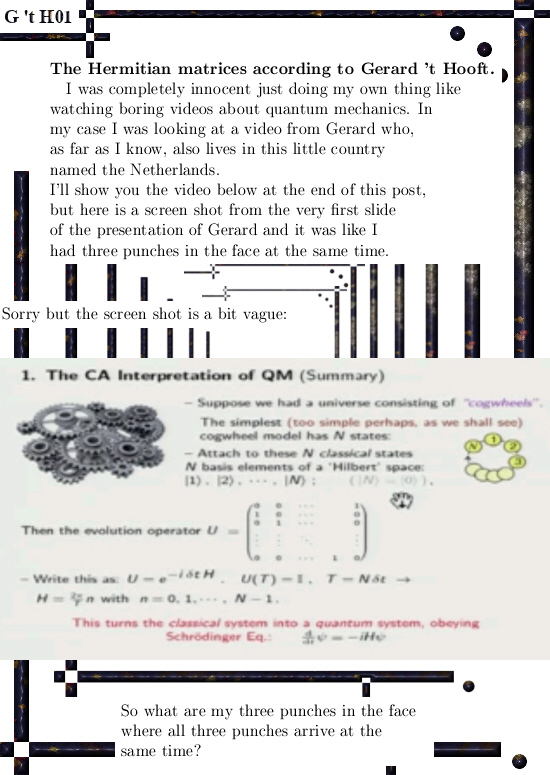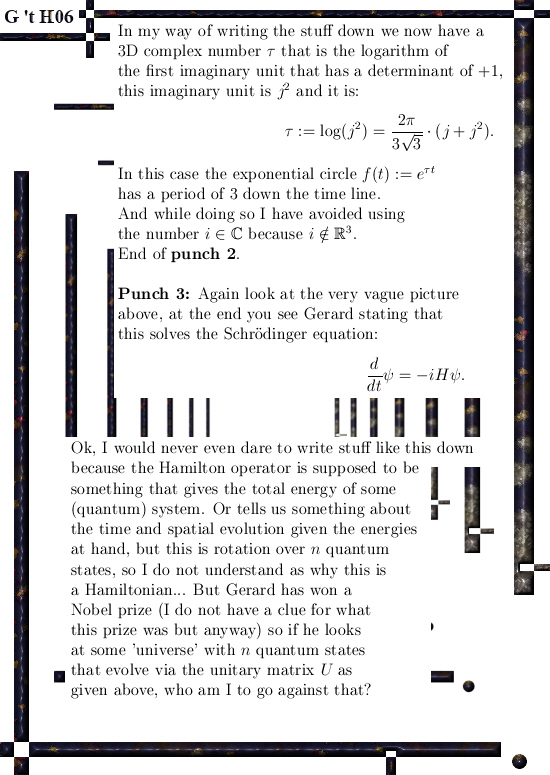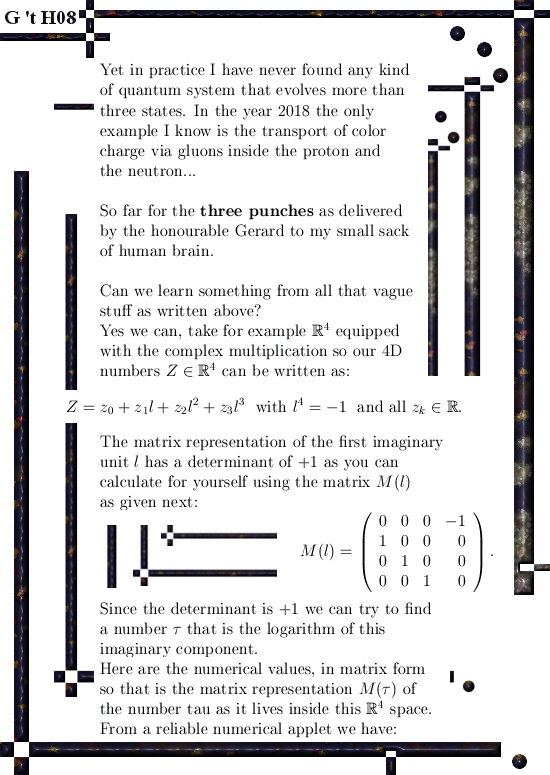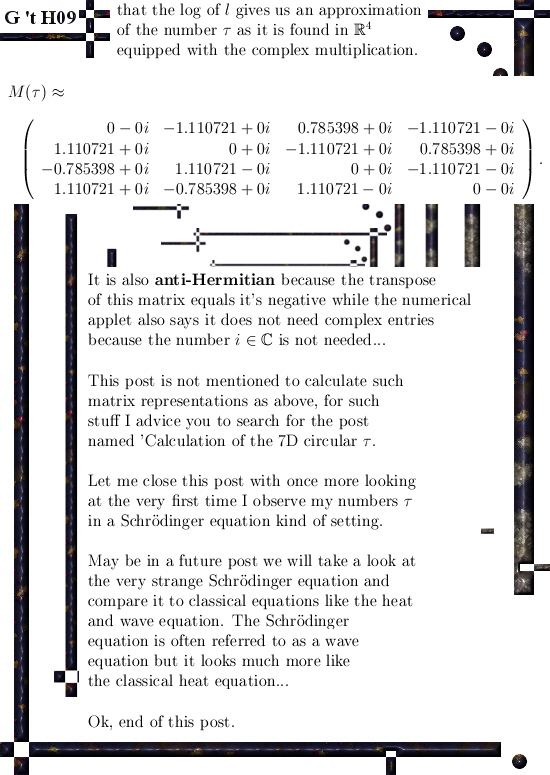For readers who have found themselves lost on what a Hermitian matrix is, here is a wiki:

Hermitian matrix
https://en.wikipedia.org/wiki/Hermitian_matrix

And for readers who have found themselves lost on finding an ‘analytic handle’ about how to calculate matrices like in picture 09, a good starter would be about the calculation of the 7D number tau:

An important calculation of the 7D number tau (circular version).

# An important calculation of the 7D number tau (circular version).

I really took the time to compose this post; basically it is not extremely difficult to understand. Everybody who once has done matrix diagonalization and is still familiar with the diverse concepts and ideas around that can understand what we are doing here.

It is the fact that it is seven dimensional that makes it hard to write down the calculations in a transparent manner. I think I have succeeded in that detail of transparency because at the end we have to multiply three of those large seven by seven matrices with each other and mostly that is asking for loosing oversight.

Luckily one of those matrices is a diagonal matrix and with a tiny trick we can avoid the bulk of the matrix calculations by calculating the conjugate of the number tau.

Just like in the complex plane where the conjugate of the number i equals -i, for tau goes the same.

Basically the numbers tau are always the logarithm of the first imaginary component. But check if the determinant is one because you can use the tau to craft an exponential curve that will go through all basis vectors with determinant one.

This post is 10 pictures long (size 550 x 775), in the beginning I use an applet for the numerical calculation of the matrix representation of the first imaginary unit in 7D space, here is the link:

Matrix logarithm calculator
http://calculator.vhex.net/calculator/linear-algebra/matrix-logarithm

Two years back in 2015 after I found the five dimensional numbers tau every now and then I typed in a higher dimensional imaginary unit and after that only staring at the screen of the computer: How to find those numbers as the log applet says…

The method as shown here can be applied in all dimensions and you now have a standard way of crafting exponential curves in all spaces you want. This method together with the modified Dirichlet kernels that provide always a parametrization of the exponential curve form a complete description.
Ok ok those modified Dirichlet kernels always have period pi while this way to calculation the log of the first imaginary unit is always related to the dimension (recall that the 7D first imaginary unit l has the property l^7 while for the complex multiplication in 7D space we have l^7 = -1), but it is very easy to fix the Dirichlet kernels to the proper period in the time domain you want.

The most difficult part of this post is in understanding the subtle choice for the eigenvalues of tau = log l, or better; choosing the eigenvalues of the matrix representation involved. That makes or breaks this method, if done wrong you end up with a giant pile of nonsense…

Have fun reading it and if this is your first time you encounter those matrices with all these roots of unity in them, take your time and once more: take your time.
If you have never seen a matrix like that it is very hard to understand this post in only one reading…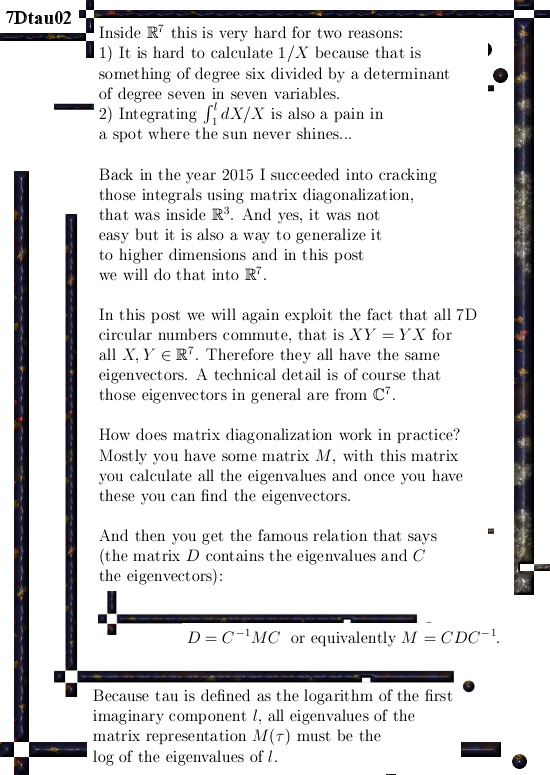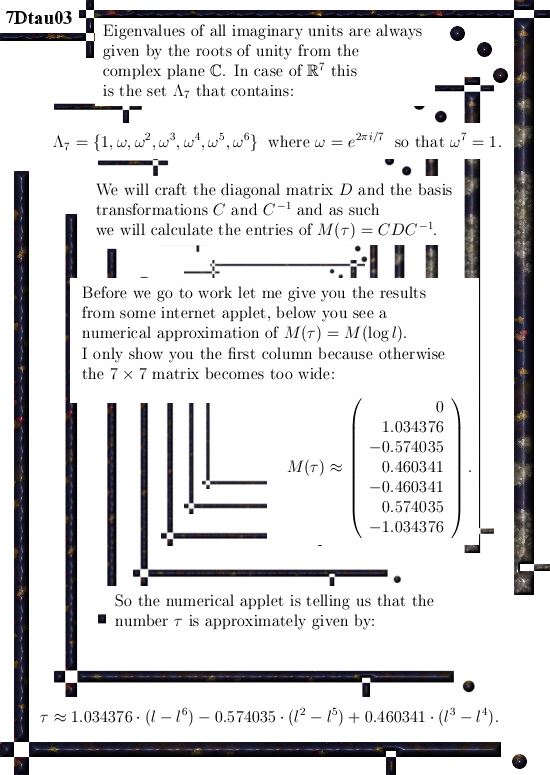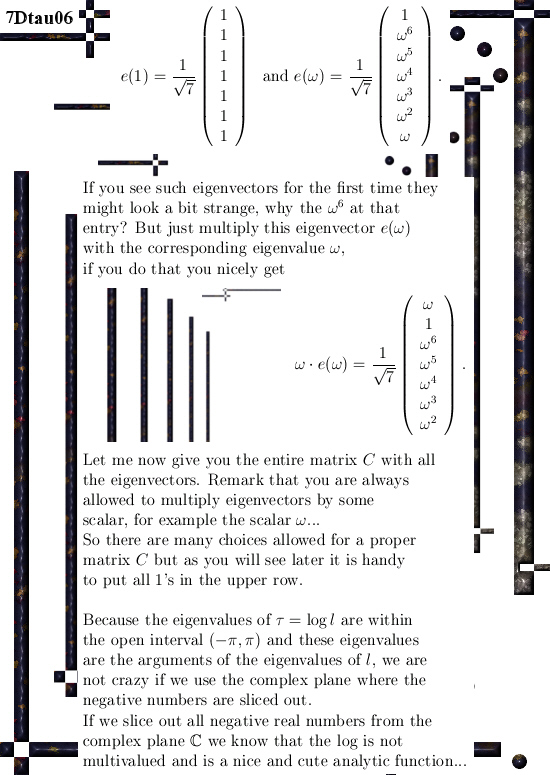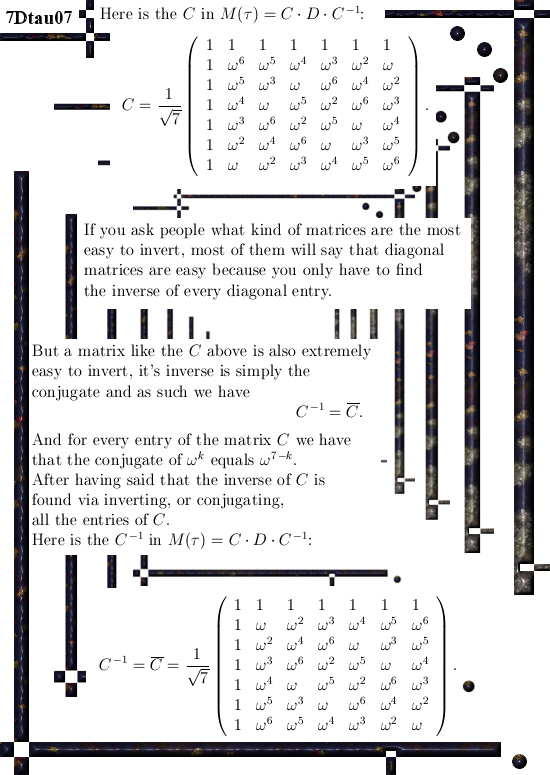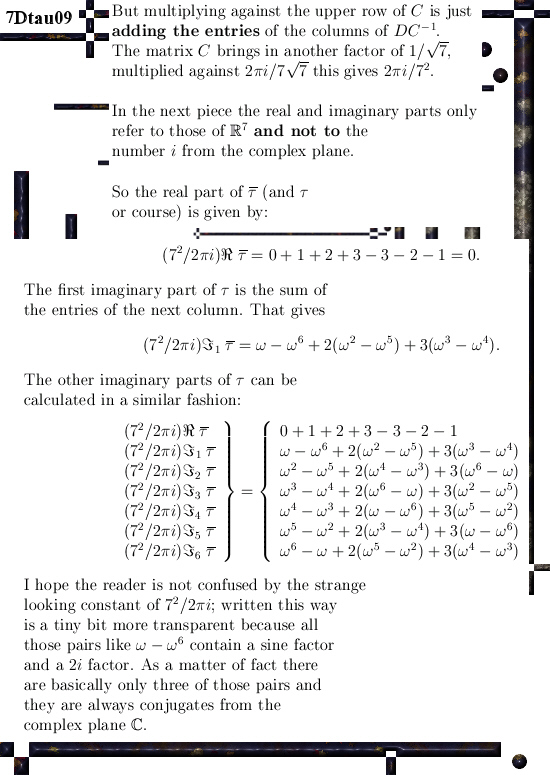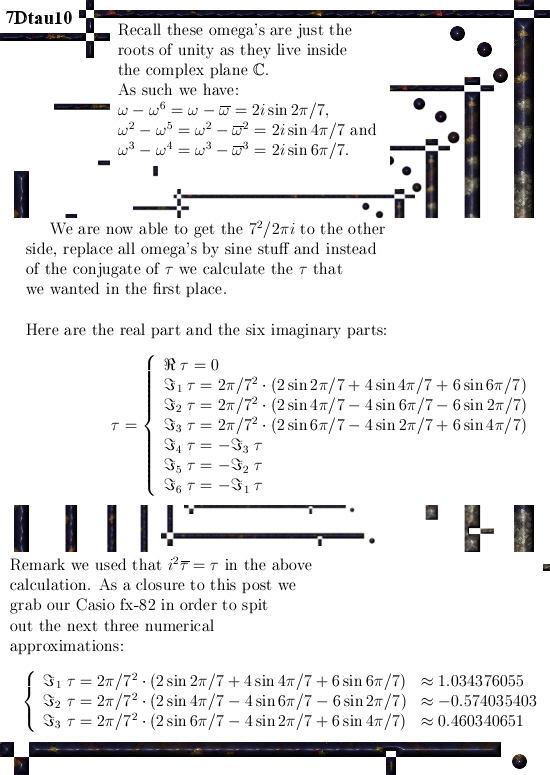I am glad all that staring to those numerical values is over and we have the onset of analytical understanding of how they are in terms of the angle 2 pi over 7.
The result is far from trivial; with the three or five dimensional case you can use other ways but the higher the dimension becomes the harder it gets.

This method that strongly relies on finding the correct diagonal matrix only becomes more difficult because the size of the matrices grows. So only the execution of the calculation becomes more cumbersome, the basic idea stays the same.

__________

I have no idea what the next post is going to be, may be a bit of magnetism because a few days back I got some good idea in explaining the behaviour of solar plasma included all those giant rings that shoot up and land in another spot of the sun.

And we also have those results from the Juno mission to Jupiter where the electrons also come from Jupiter itself without the guidance of electrical fields. But in the preprint archive I still cannot find only one work about it, that might be logical because often people do not write about stuff they don’t understand…

Ok, that was it. I hope you liked it & see ya around.